﻿ 基于三支决策的序列数据代价敏感分类算法
«上一篇文章快速检索 高级检索

 智能系统学报2019, Vol. 14Issue (6): 1255-1261  DOI: 10.11992/tis.2019050490

### 引用本文LIU Mulei, XU Feifei. A sequence data, cost-sensitive classification algorithm based on three-way decisions[J]. CAAI Transactions on Intelligent Systems, 2019, 14(6): 1255-1261. DOI: 10.11992/tis.201905049.### 文章历史

A sequence data, cost-sensitive classification algorithm based on three-way decisions
LIU Mulei , XU FeifeiSchool of Computer Science and Technology, Shanghai University of Electric Power, Shanghai 200090, China
Abstract: Cost-sensitive classification is different from the general classification method, which pays more attention to the classification accuracy of high-cost categories, but tolerates the accuracy of global classification. Three-way decisions are a solution to a cost-sensitive classification problem and lack support for sequence data. Combined with the ability of the LSTM model in sequence data processing, a method for classifying sequence data a using three-way decision method (3WD) is proposed. First, a general classification of the original data was done through the LSTM network; second, an overall cost estimate was performed on the classification result of step one; finally, the high-risk result was delayed or rejected. Methods were tested on four data sets and two sets of comparative experiments were performed. Experimental results showed that the new method distinguished the classification results of the LSTM model without changing the original structure.
Key words: cost-sensitive    three-way decision    LSTM    sequence data classification    classification algorithm    high-cost categorie    cost estimate

1 相关工作 1.1 三支决策表 1 三支决策的损失函数 Tab.1 Loss function of 3WD

(P)当 ${{{P}}_{{r}}}\left( {{{X|}}\left[ {{x}} \right]} \right) \geqslant \alpha$ 时， $x \in {\rm POS}\left( {\rm{X}} \right);$

(B)当 $\beta < {{{P}}_{{r}}}\left( {{{X|}}\left[ {{x}} \right]} \right) <\alpha$ 时， $x \in{\rm BND}\left( {{X}} \right);$

(N)当 ${{{P}}_{{r}}}\left( {{{X|}}\left[ {{x}} \right]} \right) \leqslant \beta$ 时， $x \in {\rm NEG}\left( {{X}} \right);$

 ${\rm{\alpha }} = \frac{{{{\rm{{\textit{λ}} }}_{{\rm{PN}}}} - {{\rm{{\textit{λ}} }}_{{\rm{BN}}}}}}{{\left( {{{\rm{{\textit{λ}} }}_{{\rm{PN}}}} - {{\rm{{\textit{λ}} }}_{{\rm{BN}}}}} \right) + \left( {{{\rm{{\textit{λ}} }}_{{\rm{BP}}}} - {{\rm{{\textit{λ}} }}_{{\rm{PP}}}}} \right)}}$ (1)
 ${\rm{\beta }} = \frac{{{{\rm{\textit{λ} }}_{{\rm{BN}}}} - {{\rm{\textit{λ} }}_{{\rm{NN}}}}}}{{\left( {{{\rm{\textit{λ} }}_{{\rm{BN}}}} - {{\rm{\textit{λ} }}_{{\rm{NN}}}}} \right) + \left( {{{\rm{\textit{λ} }}_{{\rm{NP}}}} - {{\rm{\textit{λ} }}_{{\rm{NP}}}}} \right)}}$ (2)

 $0 \leqslant {\rm{\beta }} < \alpha \leqslant 1$ (3)
1.2 长短时记忆网络

LSTM是由Hoehreiterhe与Schmiduhber于1997年提出后经过大量的改进，目前被广泛应用，成为目前处理序列与时序问题上的热门方案。LSTM是由一般的RNN改进而来。LSTM与一般的RNN的主要区别是在LSTM中的神经元不再是由单纯的神经元组成而是由4个功能不同的门来共同作用。其中包括了输入门、输出门、状态门，以及遗忘门。具体的结构如图1所示。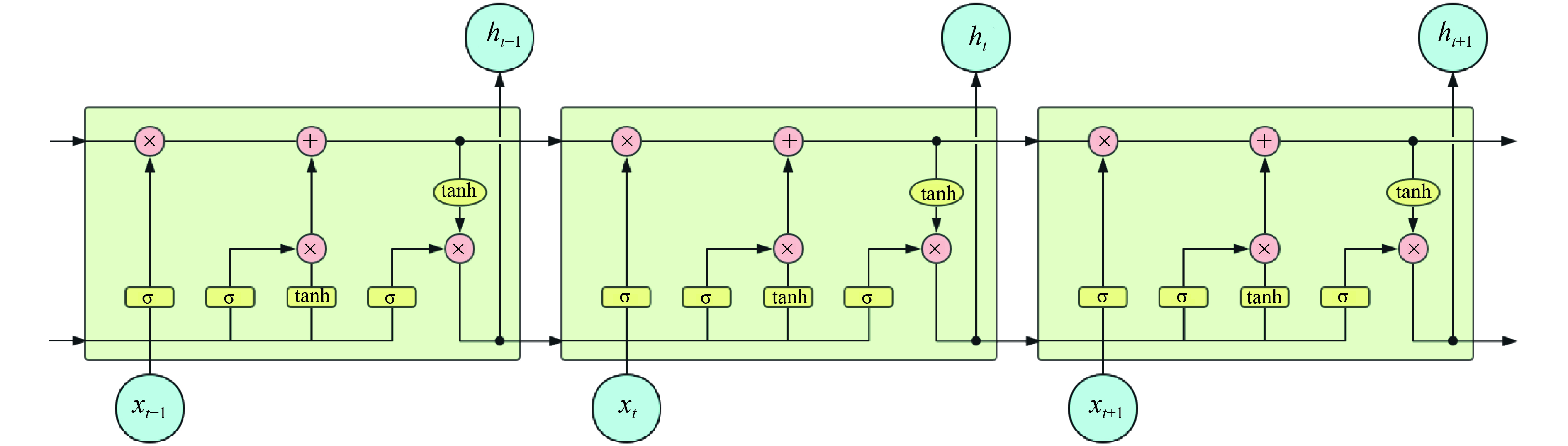Download: 图 1 LSTM网络结构 Fig. 1 LSTM network structure

LSTM的独特结构是为了使其能够解决长期依赖问题而专门设计的。不同于RNN网络，LSTM的重复结构是由更加复杂的3个门相互连接而成。包括遗忘门、输入门与输出门。

 ${f_t} = \sigma \left( {{W_f} \cdot \left[ {{h_{t - 1}},{x_t}} \right] + {b_f}} \right)$ (4)
 ${i_t} = {\rm{\sigma }}\left( {{W_i} \cdot \left[ {{h_{t - 1}},{x_t}} \right] + {b_i}} \right)$ (5)
 $\widetilde {{C_t}} = {\rm tanh}\left( {{W_C} \cdot \left[ {{h_{t - 1}},{x_t}} \right] + {b_C}} \right)$ (6)
 ${C_t} = {f_t}{\cdot }{C_{t - 1}} + {i_t}{\cdot}\widetilde {{C_t}}$ (7)
 ${o_t} = {\rm{\sigma }}\left( {{W_o} \cdot \left[ {{h_{t - 1}},{x_t}} \right] + {b_o}} \right)$ (8)
 ${h_t} = {o_t}{\cdot}{\rm tanh}\left( {{C_t}} \right)$ (9)

1.3 代价敏感分类

 $L\left( {x,i} \right) = \mathop \sum \limits_j P\left( {j{\rm{|}}x} \right)c\left( {i,j} \right)$ (10)

2 基于LSTM的三支决策分类算法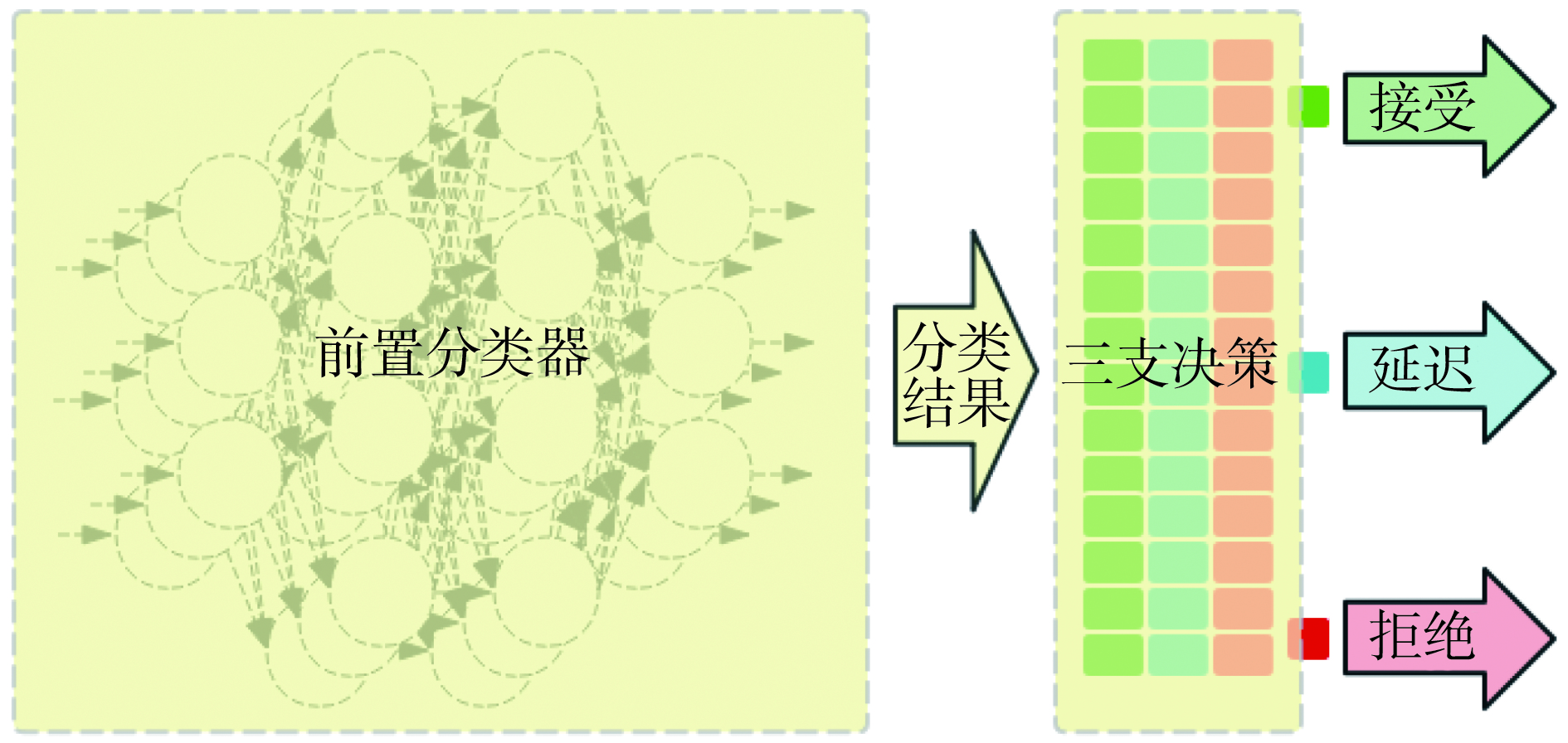Download: 图 2 基于LSTM的三支决策算法流程 Fig. 2 Flow of 3WD based on LSTM

2.1 前置分类器

2.2 三支决策

 $\begin{array}{l} \left( {\rm Pli} \right){\rm IF}\;\;{\rm Pr}\left( {X{\rm{|}}{u_i}} \right) \geqslant {\alpha _i},\;\;{\rm THEN}\;\;{u_i} \in {\rm POS}\left( X \right)\\ \left( {\rm Bli} \right)\;{\rm IF}\;{\beta _i} < Pr\left( {X{\rm{|}}{u_i}} \right) < {\alpha _i},\;{\rm THEN}\;{u_i} \in {\rm BND}\left( X \right)\\ \left( {\rm Nli} \right)\;{\rm IF}\;{\rm Pr}\left( {X{\rm{|}}{u_i}} \right) \leqslant {\beta _i},\;{\rm THEN}\;{u_i} \in {\rm NEG}\left( X \right) \end{array}$
2.3 算法概述

BEGIN：

1) 　输入 $f$ $t:$ 分类特征，分类表

2) 　 $t' =$ 由LSTM模型预测或分类数据 $t$

3) 　输入 $v:$ 代价函数表

4) 　计算边界 $\alpha$ $\beta$

5) 　FOR 样本 $i$ IN $t'$ :

计算分类概率 ${p_i}$

IF ${p_i} \geqslant \alpha$ :

$i \in {\rm pos}$

ELSE IF $\alpha > {\rm pi} \geqslant \beta$ :

$i \in {\rm bnd}$

ELSE:

$i \in {\rm neg}$

6) 　 $d=$ 计算整体代价

7) 　IF $d >$ 目标值 $d'$ :

GOTO 2

END

3 实验与结果

PM2.5数据集来自于UCI数据库，该数据集记录了从2010年1月1日至2014年12月31日北京市的空气质量指数和气象数据。数据集为时间序列数据，特征为连续特征，任务可作为分类或回归任务。数据一共43 824条记录，特征共13个，部分数据缺失。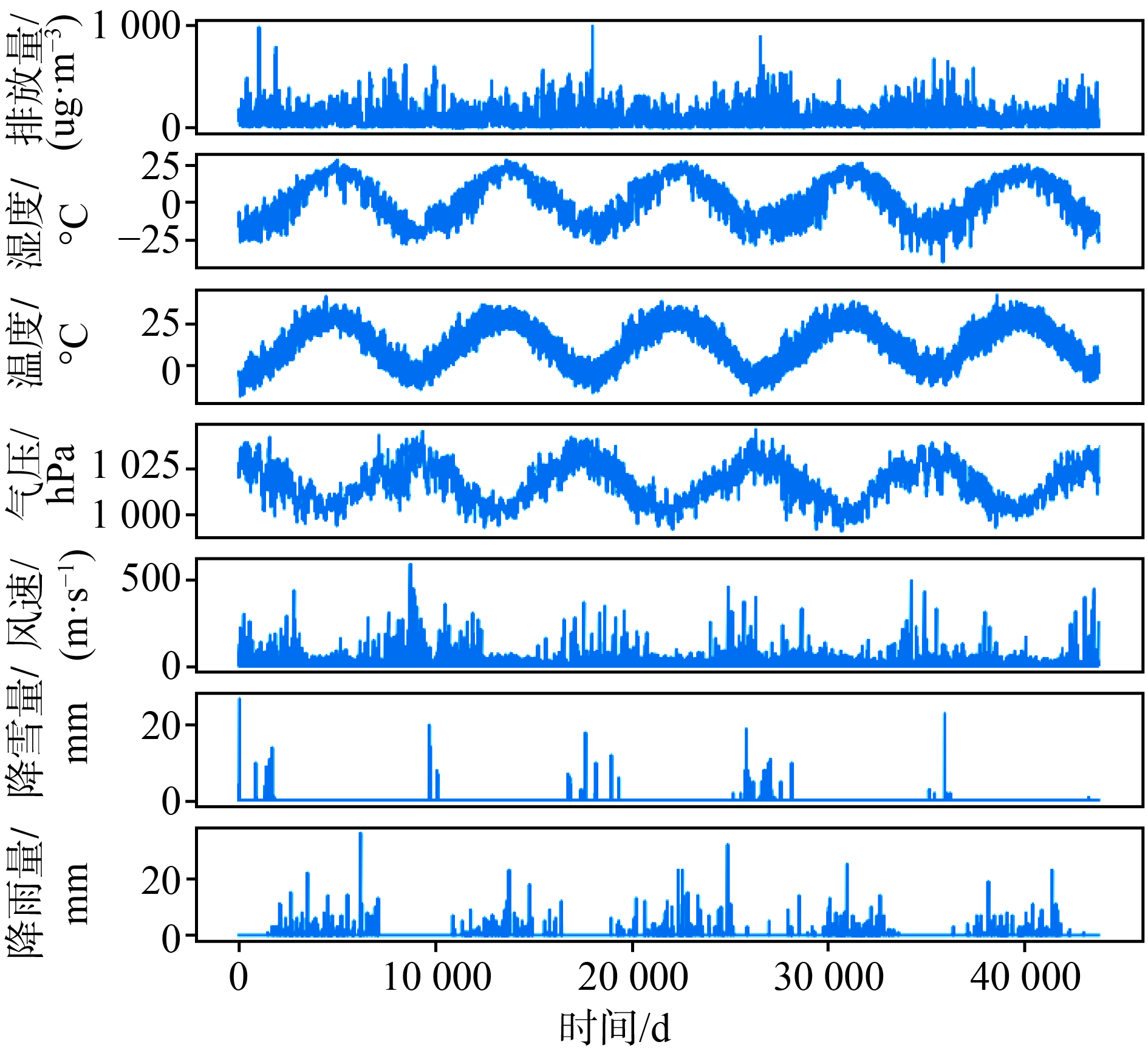Download: 图 3 原始数据集中的特征分布 Fig. 3 Frequency of features in this dataset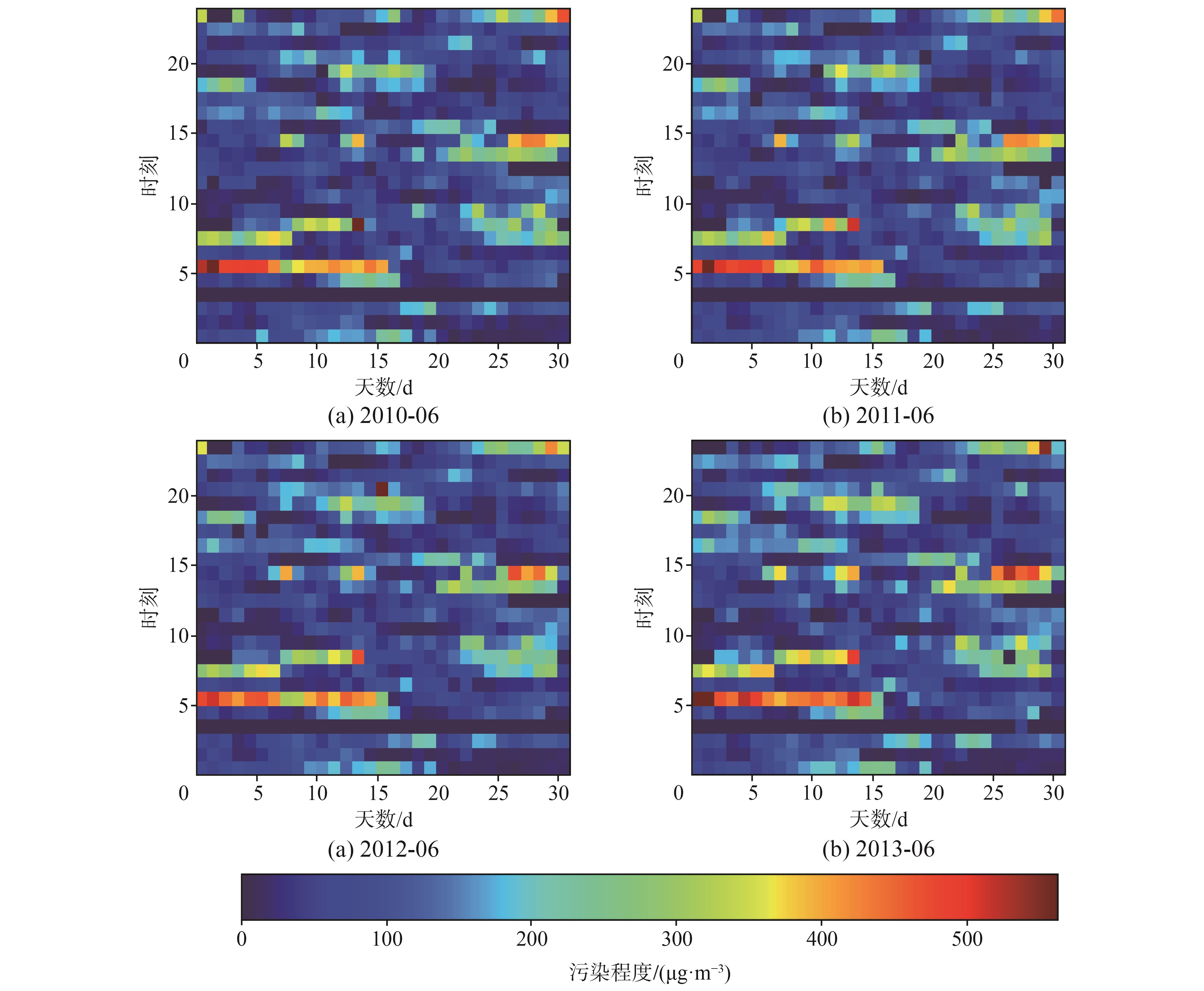Download: 图 4 原数据集中分类结果与时间的变化关系 Fig. 4 Relations between classify result and time change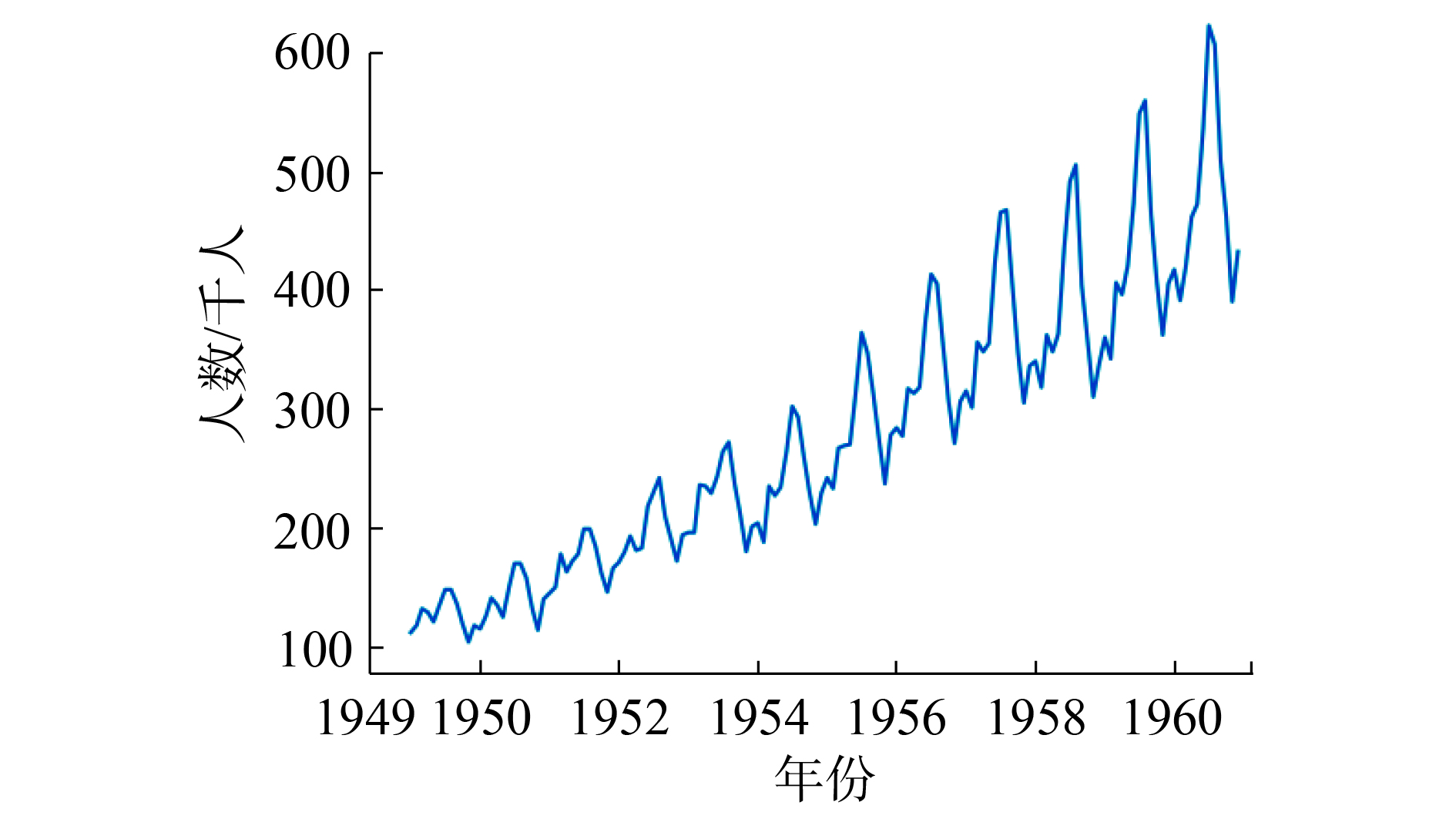Download: 图 5 1949—1960年国际航班旅客人数 Fig. 5 Number of international airline traveler between 1949—1960
3.1 PM2.5数据集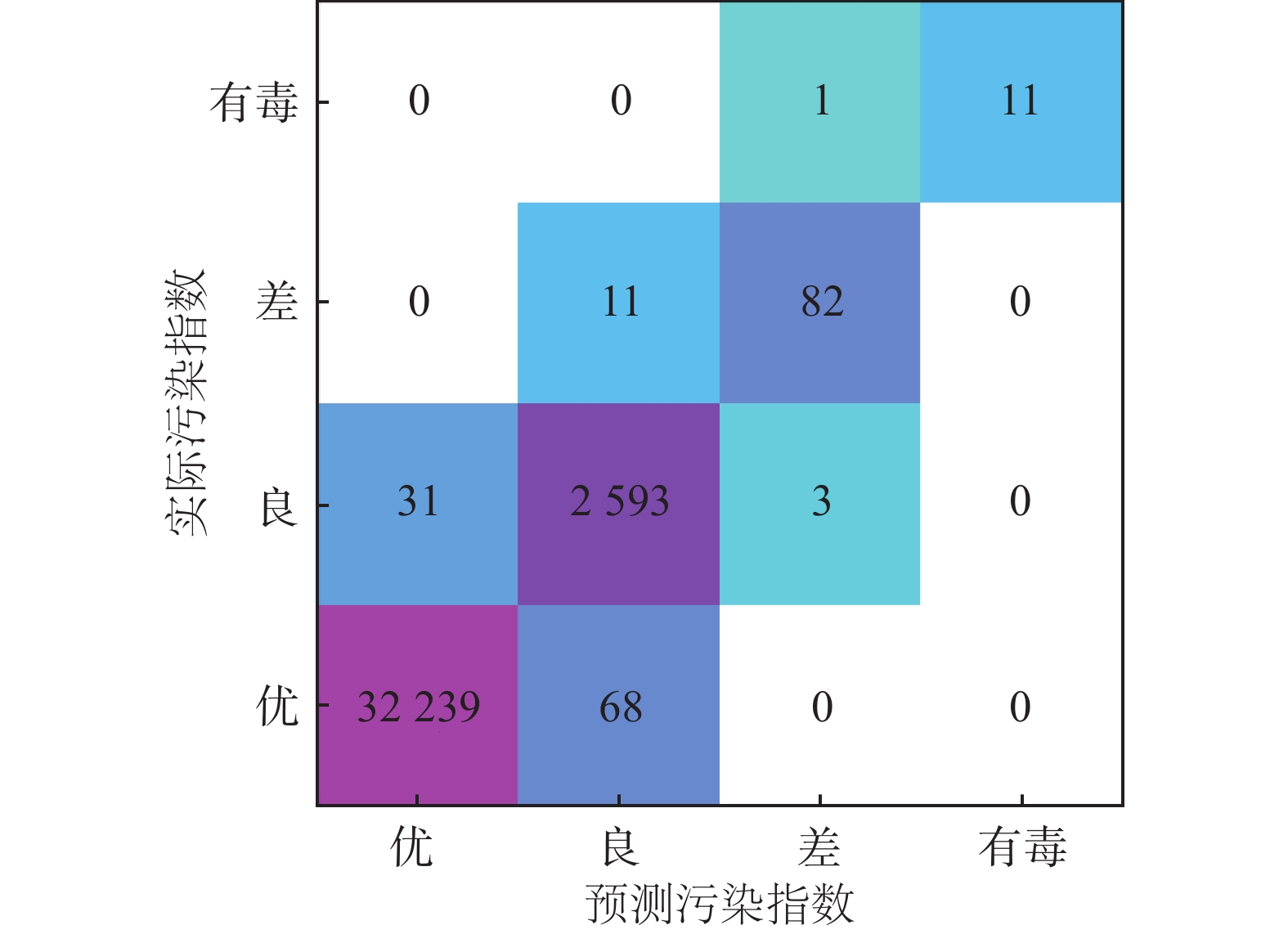Download: 图 6 分类结果的混淆矩阵 Fig. 6 Confusion matrix of classification result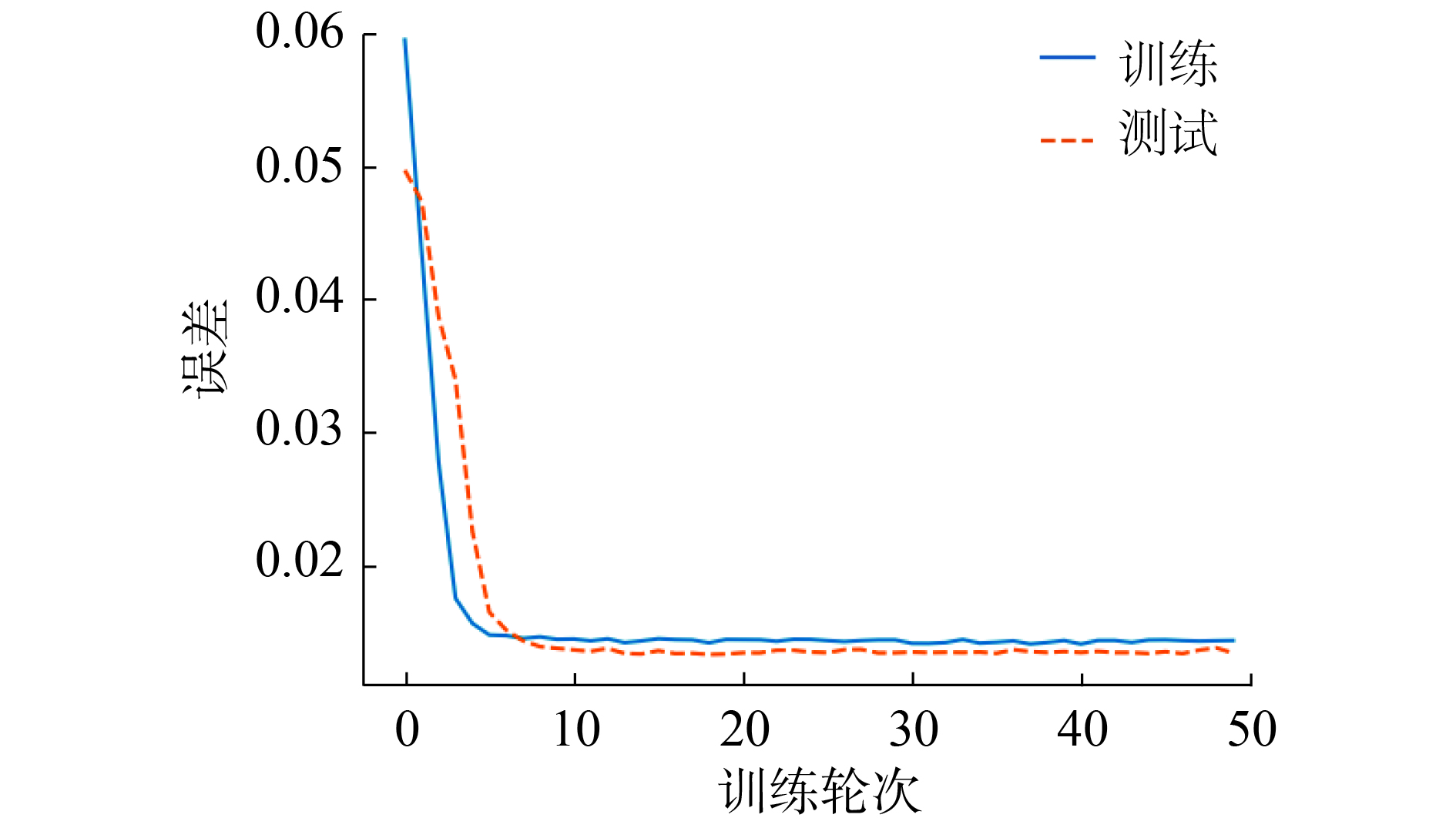Download: 图 7 LSTM训练损失 Fig. 7 Loss of LSTM training

 ${\rm{\lambda }} = \mathop \sum \limits_{{i}} {{\rm{\lambda }}_{{{ix}}}}{{{w}}_{{{ix}}}} + {{b}}$ (11)表 2 使用三支决策的分类代价与未使用三支决策的分类代价对比 Tab.2 Compara of cost of classification between 3WD and non-3WD
3.2 国际旅行旅客人数数据集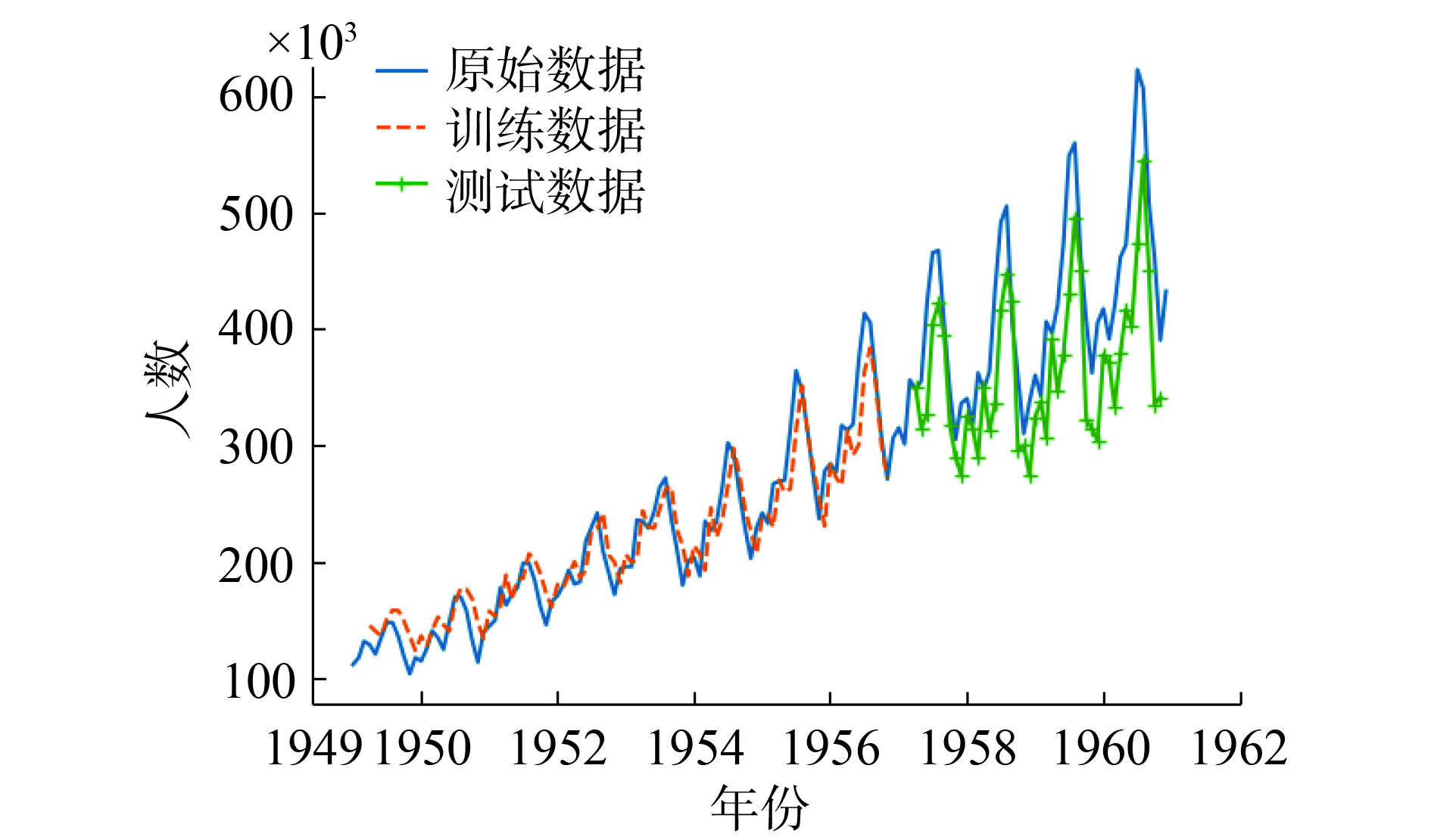Download: 图 8 经过前置分类器的预测数据 Fig. 8 Predict data after preprocessing

 $\textit{λ} = \mathop \sum \limits_{i \in \left\{ {P,B,N} \right\},j \in \left\{ {X,\neg X} \right\}} {\textit{λ} _{i,j}}{w_{i,j}} + b$ (12)

 ${{\rm{\textit{λ} }}_{{{i}},{{j}}}} \sim {{f}}\left( {{\hat{\rm \theta }},{{{t}}_{{n}}}} \right)$ (13)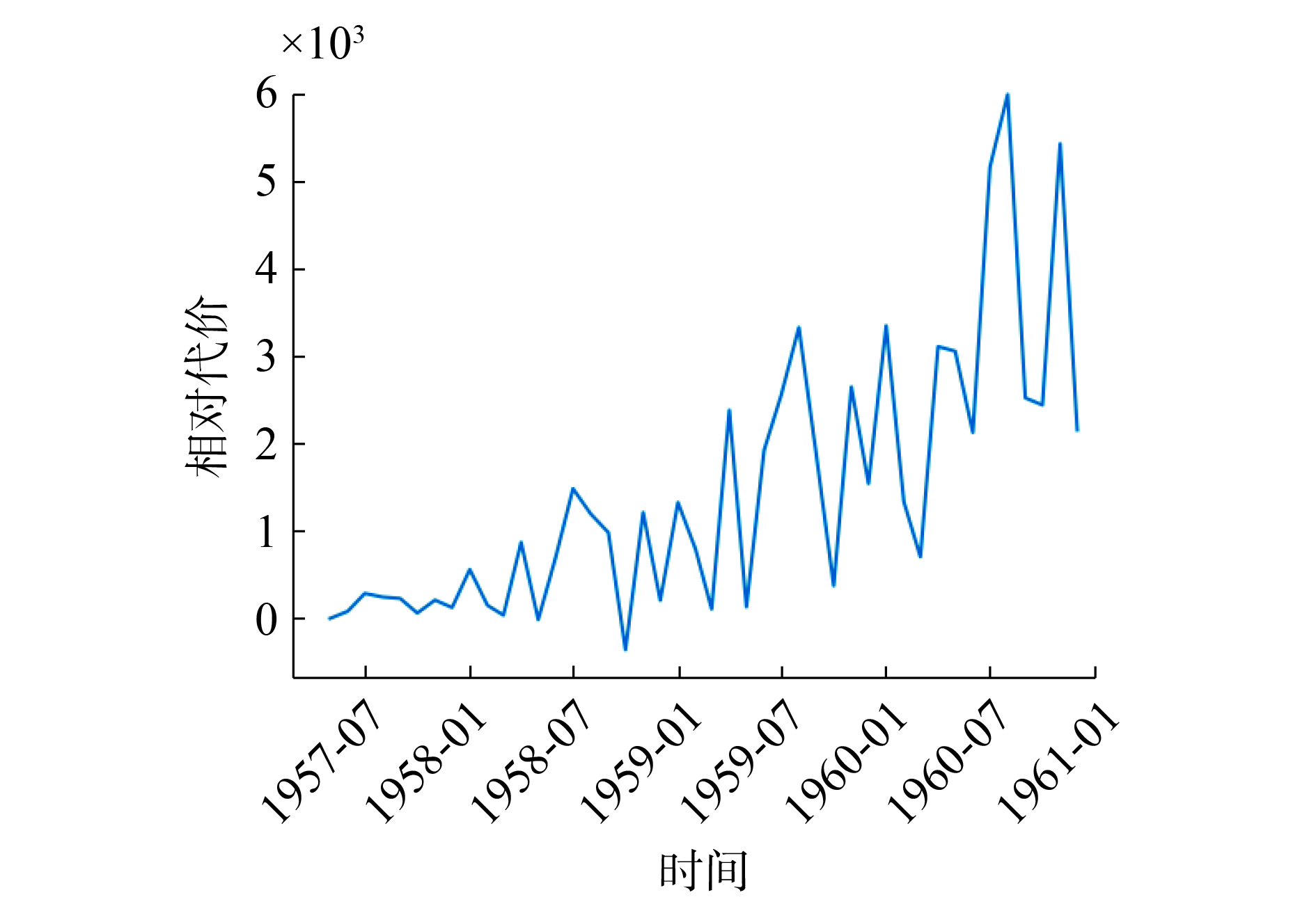Download: 图 9 随 ${t_n}$ 而代价越来越大的判断曲线 ${\textit{λ} _t}$ Fig. 9 As ${{{t}}_{{n}}}$ increases, the prediction results become more and more inaccurate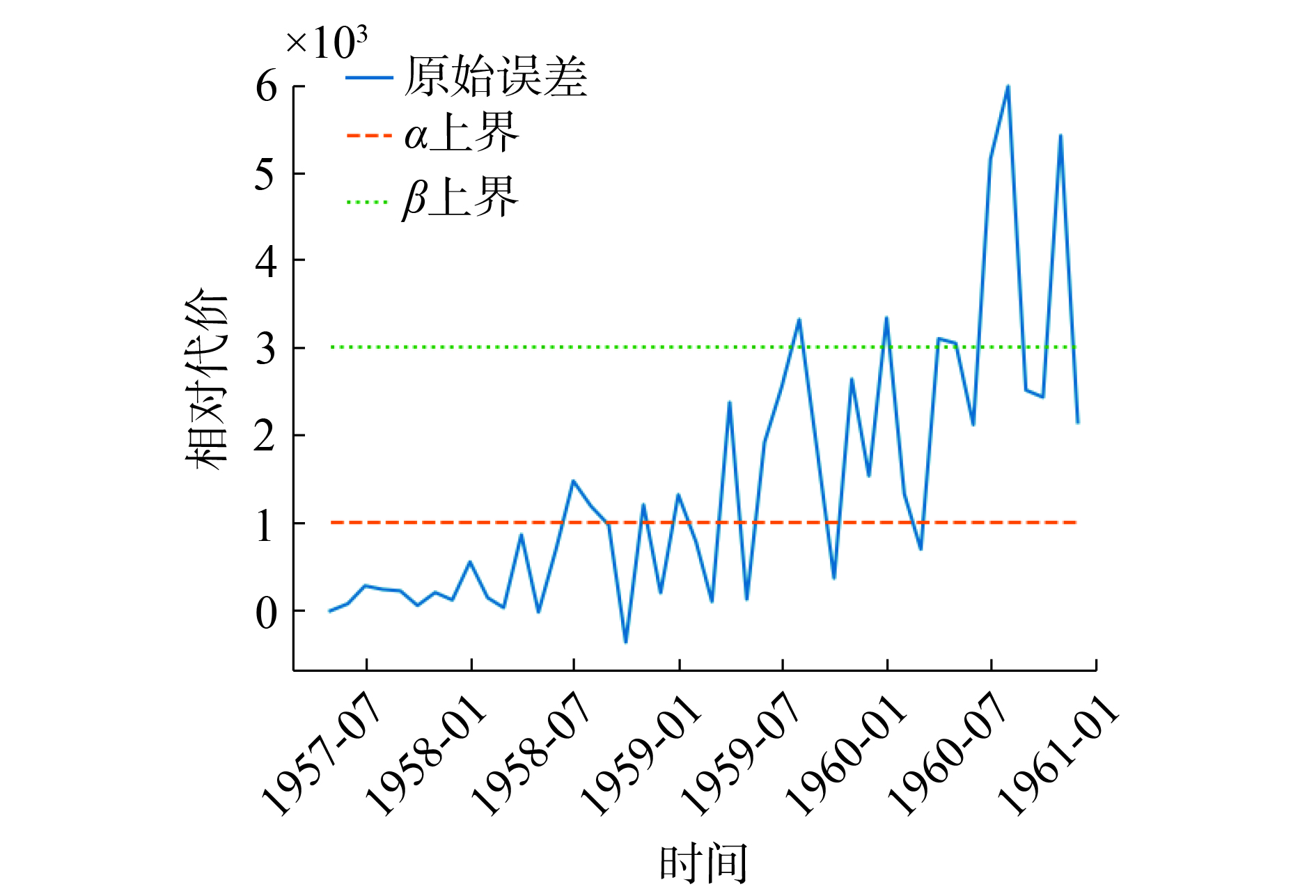Download: 图 10 代价判断决定的三支决策结果 Fig. 10 Discarding costly predictions given by 3WD
4 结束语

  KARIM F, MAJUMDAR S, DARABI H, et al. LSTM fully convolutional networks for time series classification[J]. IEEE access, 2018, 6: 1662-1669. DOI:10.1109/ACCESS.2017.2779939 (0)  KARIM F, MAJUMDAR S, DARABI H, et al. Multivariate LSTM-FCNs for time series classification[J]. Neural networks, 2019, 116: 237-245. DOI:10.1016/j.neunet.2019.04.014 (0)  KHAN S H, HAYAT M, BENNAMOUN M, et al. Cost-sensitive learning of deep feature representations from imbalanced data[J]. IEEE transactions on neural networks and learning systems, 2018, 29(8): 3573-3587. DOI:10.1109/TNNLS.2017.2732482 (0)  FERNÁNDEZ A, GARCÍA S, GALAR M, et al. Cost-sensitive learning[M]//FERNÁNDEZ A, GARCÍA S, GALAR M, et al. Learning from Imbalanced Data Sets. Cham: Springer, 2018: 63-78. (0)  YAN Ke, MA Lulu, DAI Yuting, et al. Cost-sensitive and sequential feature selection for chiller fault detection and diagnosis[J]. International journal of refrigeration, 2018, 86: 401-409. DOI:10.1016/j.ijrefrig.2017.11.003 (0)  JIANG Xinxin, PAN Shirui, LONG Guodong, et al. Cost-sensitive parallel learning framework for insurance intelligence operation[J]. IEEE transactions on industrial electronics, 2019, 66(12): 9713-9723. DOI:10.1109/TIE.2018.2873526 (0)  CHEN Jie, WU Zhongcheng, ZHANG Jun. Driving safety risk prediction using cost-sensitive with Nonnegativity-constrained Autoencoders based on imbalanced naturalistic driving data[J/OL]. IEEE transactions on intelligent transportation systems: (2019-01-17). https://ieeexplore.ieee.org/document/8617709. DOI: 10.1109/TITS.2018.2886280. (0)  YAO Yiyu. Three-way decision: an interpretation of rules in rough set theory[C]//Proceedings of the 4th International Conference on Rough Sets and Knowledge Technology. Gold Coast, Australia, 2009: 642–649. (0)  GERS F A, SCHMIDHUBER J, CUMMINS F. Learning to forget: continual prediction with LSTM[J]. Neural computation, 2000, 12(10): 2451-2471. DOI:10.1162/089976600300015015 (0)  ELKAN C. The foundations of cost-sensitive learning[C]//Proceedings of the 17th International Joint Conference of Artificial Intelligence. Morgan Kaufmann, Seattle, 2001: 973–978. (0)  LIU Xuying, ZHOU Zhihua. The influence of class imbalance on cost-sensitive learning: an empirical study[C]//Proceedings of the 6th International Conference on Data Mining. Hong Kong, China, 2006: 970–974. (0)  ZADROZNY B, LANGFORD J, ABE N. Cost-sensitive learning by cost-proportionate example weighting[C]//Proceedings of the 3rd IEEE International Conference on Data Mining. Melbourne, FL, USA, 2003: 435–442. (0)